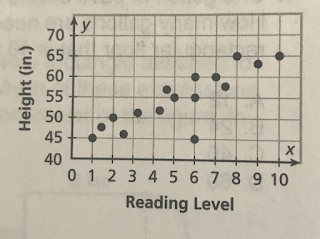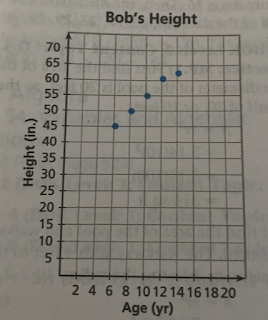## Labels

### GED Math Review: Scatter Plots

Questions:
Directions: Choose or write the answer to each question. Draw on the given graph for question 1 and 5.

Use the table of Bob's height at different ages for questions 1 and 2.

1. Make a scatter plot of Bob's data. Include a scale and a label for each axis.2. What types of associations describe the relation between Bob's height and age?

• negative, linear
• negative, nonlinear
• positive, linear
• positive, nonlinear

Use the scatter plot of the height and reading level of Ms. Banks' students for questions 3 through 5.
3. What types of associations describe the relation between height and reading level?• negative, linear
• negative, nonlinear
• positive, linear
• positive, nonlinear
4. Ms. Banks concludes that an increase in reading level causes an increase in height. Is her conclusion reasonable?
• Yes, the points cluster along a line.
• Yes, as reading level increases, the height increases.
• No, an increase in height causes an increase in reading level.
• No, association does not mean a cause-and-effect relationship.
5. Add a point that is an outlier to the graph.

1.2. positive, linear
3. positive, linear
4. No, association does not mean a cause-and-effect relationship.